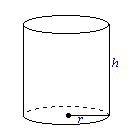The general equation for the volume of a cylindrical object is noted by the following equation.

$v = \pi r^2h$If a pizza has a radius of z and a height of a, then the volume of a pizza can be determined by the following equation:

$p_v = pi * z * z * a$